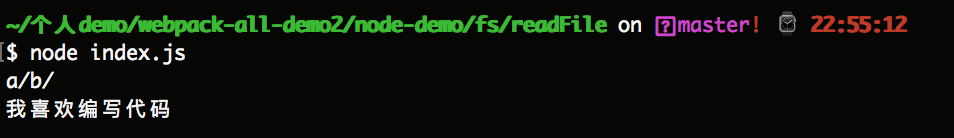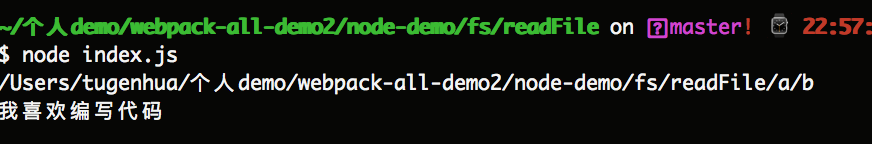## 理解Path对路径进行操作的API

2018-11-11 23:42  龙恩0707  阅读(4009)  评论(0编辑  收藏  举报

1. 解析路径字符串中的 '..' 字符串与 '.' 字符串，返回解析后的标准路径。
2. 将多个斜杠字符串转换为一个斜杠字符串，比如将 '\\' 转换为 '\'。
3. 将windows操作系统中的反斜杠字符串转换为正斜杠字符串。
4. 如果路径字符串以斜杠字符串结尾，则在转换后的完整路径字符串末尾保留该斜杠字符串。

path.normalize(path);

const fs = require('fs');

const path = require('path');

const myPath = path.normalize('.//a//b//d//../e//..//');
console.log(myPath); // 输出 a/b/

const file = fs.createReadStream(myPath + 'message.txt');
file.on('data', (data) => {
console.log(data.toString()); // 输出 我喜欢编写代码
});path.join([path1], [path2], [...]);

const fs = require('fs');

const path = require('path');

const myPath = path.join(__dirname, 'a', 'b');

console.log(myPath);

const file = fs.createReadStream(myPath + '/message.txt');

file.on('data', (data) => {
console.log(data.toString());
});path.dirname(p);

const path = require('path');

// 指定相对目录路径
const a = path.dirname('./a/b/c/d');
console.log(a); // 输出 ./a/b/c

// 指定相对文件路径
const b = path.dirname('./a/b/c/d/message.txt');
console.log(b); // 输出 ./a/b/c/d

// 指定绝对目录路径
const c = path.dirname('/a/b/c/d');
console.log(c); // 输出 /a/b/c

// 指定绝对文件路径
const d = path.dirname('/a/b/c/d/message.txt');
console.log(d); // 输出 /a/b/c/d

path.basename(p, [ext]);

ext是可选参数，该参数的作用是在方法返回的文件名中去除该文件的扩展名。请看如下所示的基本代码：

const path = require('path');

// 默认返回文件名 index.html
const a = path.basename('/a/b/c/d/index.html');
console.log(a); // 输出 index.html

// 返回index.html后，去除.html扩展名，因此会返回 index
const b = path.basename('./a/b/c/d/index.html', '.html');
console.log(b); // 输出 index

// 返回index.html后，去除html的扩展名，因此会返回 index.
const c = path.basename('./a/b/c/d/index.html', 'html');
console.log(c); // 输出 index.

// 如果扩展名不存在的话，什么都不去除
const d = path.basename('./a/b/c/d/index.html', 'ejx');
console.log(d); // 输出 index.html

path.extname(p);

const path = require('path');

const a = path.extname('/a/index.html');
console.log(a); // 输出 '.html'

const b = path.extname('/a/index.');
console.log(b); // 输出 '.'

const c = path.extname('/a/index');
console.log(c); // 输出 ''